# Binary Subtraction (How To Guide With Rules And Examples)

## How to Subtract Binary Numbers

Like binary addition, binary subtraction also plays an important role in binary arithmetic as well as in digital electronics system. We have discussed the binary subtraction process briefly in this article on binary arithmetic. But here we will focus on the process of binary subtraction – i.e. how to subtract binary numbers.

Binary addition, binary subtraction, binary multiplication and binary division are the four types of arithmetic operations that occur in the binary arithmetic.

When adding binary numbers, there are four points or steps to remember before proceeding through the operation. These are: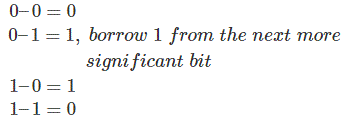As there are only two digits in binary number system 0 and 1 that’s why these four steps are able to describe all the operations of binary subtraction.

Now we will discuss the process elaborately with the help of a few examples.

Suppose, A = 10101100 and B = 1010100 and we want to find out A – B.

Now implementing the rules of binary subtractionThe first step is 0 – 0 = 0 and that’s what is written in the place for result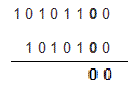Similarly again the last step is repeated as here the numbers are both 0 and from the table we know 0 – 0 = 0.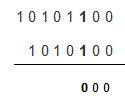From the table, we can find out that 1 – 1 = 0 and it is written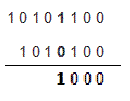The table shows that 1 – 0 = 1 and we have written exactly that in result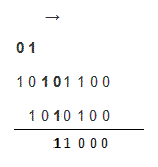Here 0 – 1 = 1 with the borrowing of 1 from the next significant bit and that’s what has been done. We will treat the next 1 as 0 in the next step as shown below.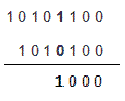As the 1 was borrowed in the previous step we are treating the 1 as 0 and the result is 0 – 0 = 0 and that is written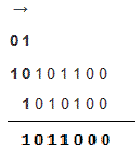Again the last 1 has been borrowed because the operation done was 0 – 1 = 1 with borrow 1 from the next most significant bit and the final result of binary subtraction, we got is written in the place of result in the final step.

Want To Learn Faster? 🎓
Get electrical articles delivered to your inbox every week.
No credit card required—it’s 100% free.